Maths is often represented as a tricky topic – by both students and adults alike! However, this little loved subject forms a fundamental part of your education, and if you can master it, you’re well on your way to succeeding academically!

For as long as you’re studying for qualifications (maths revision GCSE, A levels, degrees…) you won’t escape having to solve maths problems: starting from the basics of arithmetic, and then moving onto solving equations, algebra, geometry, and fractions problems!

Find your online maths tutor here.

Later in your studies, your maths skills will let you adopt a problem-solving mindset and improve your mental faculties, helping you succeed at the task you’re completing, regardless of whether or not it’s directly related to maths!

With all of this in mind, it’s time to revisit one of the key areas in maths that you’ll encounter time and time again: solving equations!

With your calculator to hand and a little determination, you will make it to the end of this article on how to solve an equation. By the time we’re finished, amongst other things, you’ll be able to tackle your next maths problem with ease, whether it involves polynomials, linear equations, factoring or anything in between!

## What is an Equation?

Just like in other areas, when we’re dealing with solving systems of equations and graphing them, it’s important to understand the terminology being used.

Your maths teacher will definitely tell you that knowing the definition of mathematical terms is essential if you want to progress in maths – and they’re not wrong!

So – what is an equation? According to the Larousse dictionary, it’s this:

An equality which hasn’t been solved for certain variables or unknowns

Right off the bat from the dictionary definition, the terms “variables” and “unknowns” have appeared – and they’re not going to disappear until we’re finished solving our equation! A variable is just a fancy word for substituting one value for another: if you’ve ever done any algebra at all, you’re probably familiar with the use of “x” – this is your variable. An unknown is usually a variable we don’t know the value for. This is what we’re trying to find out!

If this seems a bit technical for you, here’s a definition you’re more likely to hear in a classroom:

An equation is an equality which consists of a variable (often called ‘x’). This variable must be found to know how solve the problem

So, you now have a working knowledge of all the concepts you’ll need to start solving equations:

• An equality between two algebraic expressions
• One or more unknowns to find
• A variable called ‘x’
• Used to solve problems

Whether you’re looking at a linear equation, a quadratic equation or systems of equations, you should take the same steps to factorise, remove coefficients, and solve them.

## Skills Needed to Solve Maths Equations

To succeed at solving an equation, there are certain skills relevant to your study of mathematics, as well as the famous “problem-solving attitude” which we acquire throughout our academic careers and which helps us become competent mathematicians.

### Problem-solving attitude

A certain apprehension about mathematics is why a large number of students and adults don’t see a use for maths in their daily lives, and think of it as solely an academic subject.

In reality, maths forms an integral part of our day-to-day lives, even if you rarely count on it yourself. From food, to buying a house, to opening a bank account, to studying: maths is everywhere in our lives; whether we’re adding, multiplying, subtracting, or even solving equations without realising!

Your maths teacher at school (or your private maths tutor) will give you the skills that will serve you every day, for the rest of your life.

Here are some aspects that are key to adopting this problem-solving attitude:

#### Being thorough

You must be thorough and follow through with all the key steps when tackling a mathematical problem: particularly one including equations. When you find yourself faced with maths problems, or an exam, you must be precise, and act methodically and logically.

#### Have a good memory

Mathematicians have to work with their memory! If you practise regularly, you’ll be able to make the link between the topics you’ve studied in class, and their application in solving a problem given to you. Tackling a variety of problems means something like a problem you’ve seen before is more likely to come up, and with your prior experience, it’ll be much easier to solve.

#### Be organised!

To solve an equation, you need to follow a set of stages. Being organised in your work environment, and your approach to problems, will allow you to overcome each maths problem put in front of you without panicking.

## When do we Learn about Maths Equations?

During primary school, we learn how to count, and familiarise ourselves with mental maths. We start to add numbers, subtract them, and multiply them: the basics of arithmetic. We may even learn the basics of graphing - these teachings provide us with a foundation in mathematics, and the skills we will need to begin solving equations later on.

It’s usually in secondary school that linear equations slowly start to creep into the curriculum. In year 7, we learn to solve simple equations, normally with one unknown. Here’s an example of an equation you might tackle around this time: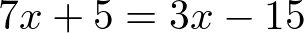Here, you must find the unknown: ‘x’.

By the time you get to year 8 and 9, fractions and negative numbers start appearing in equations: preparing you for GCSEs and A levels. The equations can now look something like this: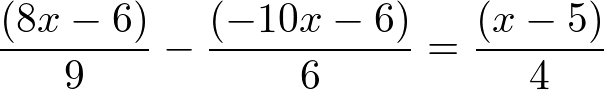At GCSE and A level, the calibre of maths problems increases, and you have to solve more complex equations, including systems of equations, by using a variety of techniques: completing the square, factoring, substitution, and finding the intercept on graphs - to name but a few! If you do some further study in maths at A level, you will also encounter topics like complex numbers.

The types of problem don’t stop there, though: if you decide to study a maths or science degree at university, you’ll meet even more difficult problems!

Sometimes you will encounter one step equations, and you might be able to solve some of the equations below like this, but you’ll often encounter trickier multi-step equations which will test your knowledge of several mathematical areas: perhaps your graphing calculator knowledge, your knowledge of slopes, or how to solve simultaneous equations by elimination or substitution!

## Solving a Linear Equation

Linear equations are likely the first type of equation you’ll encounter during your studies, and are usually the easiest to solve.

Essentially, solving a linear equation only requires the four kinds of calculation we’ve been familiar with since primary school: addition, subtraction, multiplication and division.

If you want to solve a linear equation with one unknown, your goal is simple: you must find the value of ‘x’. This is usually called “solving for 'x'” in maths lingo.

For a simple equation, you can take the following steps:

• Isolate the unknown (usually ‘x’) on one side of the equation

• Group any common terms together

• Divide through so that any coefficients of ‘x’ are removed

To give you an example, here are the steps you would take for the equation...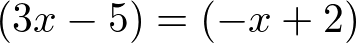Moving 'x' onto one side of the equation: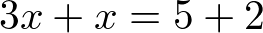‘x’ has been isolated on one side of the equation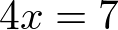We’ve grouped common terms together - this is known as collecting like terms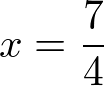We’ve removed the coefficient of ‘x’ by dividing both sides by 4.

Congratulations! You now have the skills required to solve one of the most famous linear equations in the history of mathematics: the epitaph of the mathematician Diophantus of Alexandria.

The epitaph gives an equation, and the solution to this equation is the age of Diophantus when he died. The tomb dates from the 3rd century!

Being able to solve linear equations is the cornerstone of your equations knowledge, and you must practise it again and again until you’re completely sound on the subject. Better get started!

## Solving a Factorised Equation

Factorised equations are a form of polynomial equations. and you will often see them as quadratic equations (at least up to AS level!).

Essentially, the equation takes the form: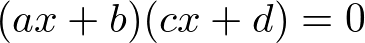‘x’ is always the unknown variable, while a,b,c, and d are numbers given in the problem. During your maths lessons, your teacher has probably told you something like this:

A product of factors is zero if, and only if, at least one of the factors is zero.

To check this is true, you must solve every equation with a factor of ‘x’ in it!

We can’t repeat this enough: the best way of learning to solve equations is to practise!

Here’s an example of a factorised equation, with its solution: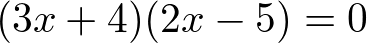The two factors correspond to the two expressions in parentheses. Just so we’re clear on the terminology, the “coefficients of 'x'” that we spoke about earlier are 3 and 2, in this example. To solve it, we must consider both sets of parentheses. We’ll go left to right, and once again we’ll solve for our unknown ‘x’: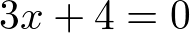(because our equation must be equal to 0 for the product of the factors to equal 0)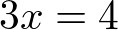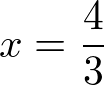And the other set of parentheses...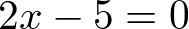(because our equation must be equal to 0 for the product of the factors to equal 0)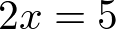And removing coefficients of 'x'...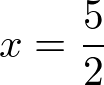Since there are two factors, that means there are two solutions for ‘x’ - this is sometimes called a solution set. We just found them both! ‘x’ can either be -4/3 or 5/2.

Being able to solve quadratic equations is essential as you progress. If you can’t solve quadratic equations, you will struggle with higher order equations. Maybe you need to refresh your knowledge on how to solve linear equations first?

## Maths Equations with Fractions

We have a rule for equations with fractions in, too! Here’s the rule:

A fraction is zero if, and only if, its numerator is zero and its denominator is not zero.

You could be faced with an equation in the following form: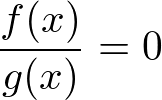To solve an equation like this with a fraction in, you should:

• Exclude forbidden values: this means values which cancel out the denominator

• Simplify everything so it’s over the same denominator

• Put everything onto one side of the equation, so the other side is equal to zero

• Solve the equation

• Verify that the values you have aren’t forbidden values

Here’s an example of solving an equation with fractions in. We’ll be using a technique called cross products to remove the fractions altogether. We start with this equation: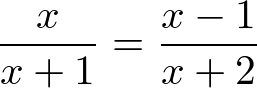Let's start by removing the fractions: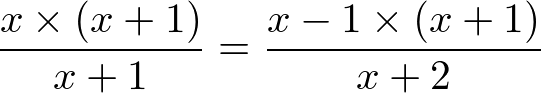Just like in our linear equation example, I multiplied both sides by (x+1). This means that on the left hand side, I now multiply by (x+1) and then immediately divide by (x+1). And just like in our previous example, that means they cancel each other out, and I can remove them from the equation altogether: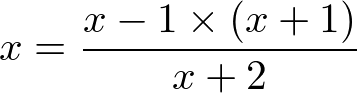So, it's starting to look a lot nicer already: I've now got a single 'x' on one side, removed one fraction, and we're well on our way to solving this problem. Let's also get rid of the fraction on the right hand side, using the same method:Removing the fraction on the right hand side means this all simplifes to something much nicer: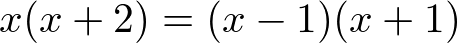Now, we need to set our equation equal to zero. We could divide through by (x-1)(x+1), but then we'd end up with a fraction again! Instead, we can expand out the contents of each set of parentheses. To do this, we multiply everything inside the parentheses by everything immediately outside of it. This is easy for the left hand side, as we only have to multiply by x: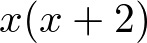becomes...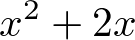Because both of these expressions are equivalent (as equalities, they are equal), we don't need to change the right hand side, as we've just rewritten the left hand side: it still means the same thing. See our GCSE Maths Revision article for more help with this.

The right hand side is a little trickier, but follows the same principle. The contents of each set of parentheses needs to be multiplied by the contents of the the other set of parentheses. Here's a diagram:The red lines show us multiplying 'x' in from the first parentheses, and the blue lines show us multiplying '-1' from the first parentheses.

This leaves us with the following expressions, starting with the 'x' in the first bracket: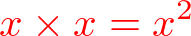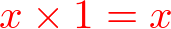And now multiplying '-1' in the first bracket: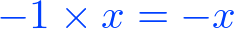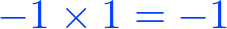So those are all the terms of the brackets on the right hand side, once they've been expanded. Now we can combine those into one expression, which represents the right hand side of the original equation: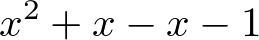Let's put these two expressions back into our original equation: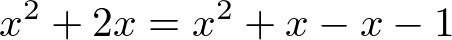Again, this is exactly the same as what we started with, we've just removed the factored parentheses. Next, we'll put anything with an x or x^2 onto the left hand side of the equation: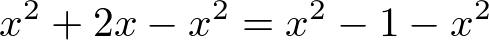And collect like terms. In the equation above, I've done the same to the left hand side as the right hand side of the equation, so we'll tidy everything up: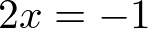So the last step should be pretty easy. We need to remove our coefficient of 'x':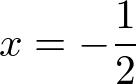-1/2 isn’t a forbidden value, so the solution is x = -1/2. Notice how despite this starting as a quadratic equation (because there’s an exponent of two on some of the ‘x’ terms), they cancel each other out, so we only have one solution.

It is possible to have an equation with no solution!

A fractional equation problem can include all of the same concepts as we’ve mentioned before, including: quadratic equations, exponents, rational and irrational numbers.

## Creating Equations to Solve Problems

Sometimes, you might be asked to form an equation for a given problem. Don’t worry, this isn’t as bad as it sounds, you’re essentially making your own word problems!

The method is simple, and you only need to make sure you’re thorough to ensure you get the correct answer:

• Read the problem several times to make sure you understand it
• Determine the unknown (or unknowns) which correspond to a number asked for by the question
• Try and rewrite the problem in mathematical terms, removing any superfluous information
• Solve the equation you just found
• Check the solution is correct
• State your equation as a solution to the problem

Sometimes you’ll be asked a question like this in the context of a geometry problem – don’t worry, the steps are the same, just make sure to brush up on your geometry lessons beforehand!

Here’s an example of a question you might be asked, where you have to form an equation from the given scenario:

“Three cousins, John, Tim and Sally, have a combined age of 60. What is the age of each person, knowing that Sally is three times as old as Tim, and that John is 10 years younger than Sally?”

In this problem, the unknowns to find are the ages of the three cousins, and this can be done with the help of some equations. See if you can solve it!

There are several options available to you if you want to improve, or perfect, your maths skills: whether that be brushing up on your equations knowledge, or learning something new about geometry!

All of the examples we looked at today dealt with rational numbers, and did not feature inequalities. However, inequalities are solved in the same way, simply replacing the equals sign with the equality sign of your choice.

Why are equations and inequalities important? They serve as the building blocks of your maths knowledge, and are essential when you begin studying calculus, and maybe even matrices!

Knowing that you can effectively deal with problems involving integers, decimals, rational numbers, and different types of equation: whether they’re exponential equations, solving quadratics, or systems of linear equations will be invaluable as you progress further into the world of maths!

Private tuition with a home maths tutor will allow you to progress. They can explain tricky topics like equations at your own pace, using methods tailored to your learning style.

Revising regularly and producing revision materials, or following maths lessons online and on YouTube, in tandem with private maths tuition will help you achieve your best in maths!

Learn how to graph functions or discover the list of unsolved maths equations.

Need a Maths teacher?5.00/5, 1 votesLoading...